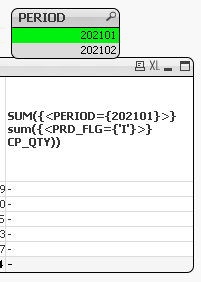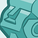# QlikView App Dev

Discussion Board for collaboration related to QlikView App Development.

Announcements
Our next Qlik Insider session will cover new key capabilities. Join us August 11th REGISTER TODAY
cancel
Showing results for
Did you mean:Contributor II

## Variable

Hi Friends

I have created following variable in QV document

vSpq=sum({<PRD_FLG={'I'}>}CP_QTY)

I want to use above variable in expression of my pivot table which is as follows

SUM({<PERIOD={\$(=Max(PERIODID))}>}\$(vSpq))Pls help me to identify the error and let me have correct expression please

3 RepliesCreator II

You cannot use nested aggregation like this.. i.e. Sum(Sum()).. look into Aggr() function to use nested aggregation.

``SUM({<{<PRD_FLG={'I'},PERIOD={\$(=Max(PERIODID))}>}CP_QTY)``Contributor II
Author

Thanks

Could you suggest me to write the expression with a variableCreator II

If you want more control / dynamic variables; look into parameterised variables!

Example for reference -

``vVariable = SUM( {<PRD_FLG={'I'},PERIOD={\$(=Max(PERIODID))}>} \$1 )``

Call parameterised variables

``\$(vVariable(CP_QTY))``Community Browser